# A Geometry Engine Interface for 'grid'

Version 4: Tuesday 14 April 2020

Version 1: original publication
Version 2: added mention of 'ggforce' and 'ggraph'
Version 3: Wednesday 01 May 2019; 'gridGeometry' now depends on 'polyclip' 1.10-0
Version 4: start adding dates to historyThis report describes a new function in 'grid' called `grobCoords` and a new package called 'gridGeometry' that combines `grobCoords` with the 'polyclip' package to provide a geometry engine interface for 'grid'.

## 1. Introduction

The 'grid' package for R (R Core Team, 2018) provides some useful tools, such as units, viewports, and layouts, that make it easy to express certain arrangements of output when drawing (e.g., 'ggplot2' plots; Wickham, 2016). However, there are many graphical results that are difficult to produce with 'grid'. A simple example is drawing an edge between two nodes on a graph or diagram, as shown below.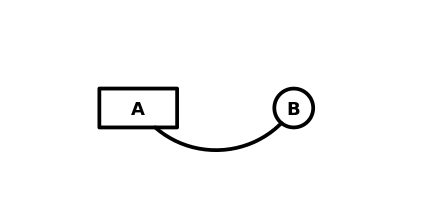To be more specific, the line between the two nodes in the above diagram is a Bezier curve that would originate and terminate at the centre of the two nodes, if it were not cut off at the node boundaries. This means that 'grid' functions like `grobX` and `grobY` are no use, because it is difficult to determine the angle of incidence of the lines to the nodes. We could use `grid.bezier`, starting at the boundaries of the nodes, but that would produce a different curve entirely, and one that would not be aiming at the node centres.

Another detail about the diagram is that the background of the nodes is transparent (as shown below). This means that the standard R graphics "painters model" cannot help us out; we cannot just draw a Bezier curve that starts and ends at the node centres and then draw opaque nodes over the top to obscure the intersection of the curve with the nodes.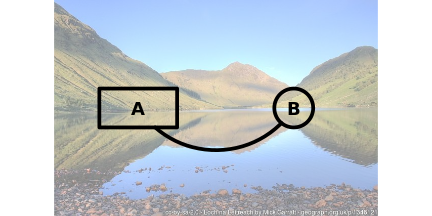The photo in the image above is © Mick Garratt (Loch na Leitreach, Sunday, 7 June, 2009, cc-by-sa/2.0). It is read into R using the 'jpeg' package (Urbanek, 2014)

One way to produce the diagram above is using "constructive geometry", where we create a shape that is difficult to describe by combining shapes that are easier to describe. In the example above, the curve between the nodes is difficult to describe, but a curve between the node centres is easy and the nodes themselves are easy. By "subtracting" the nodes from a curve between the node centres we can easily obtain the curve between the nodes. This document describes a new package for R called 'gridGeometry' (Murrell, 2019) that provides tools for performing this sort of constructive geometry with 'grid' graphics.

```library(gridGeometry)
```

The following code uses standard 'grid' functions to describe two nodes (a rectangle and a circle) at locations A and B and a Bezier curve from A to B.

```Ax <- .3
Ay <- .5
Bx <- .7
By <- .5
node1 <- rectGrob(Ax, Ay, .2, .2,
gp=gpar(lwd=5, fill=NA))
node2 <- circleGrob(Bx, By, r=.1,
gp=gpar(lwd=5, fill=NA))
line <- bezierGrob(c(Ax, .4, .6, Bx),
c(Ay, .2, .2, By),
gp=gpar(col=rgb(0,0,0,.2), lwd=5))
```

The `polyclipGrob` function from the 'gridGeometry' package can be used to create a new grob that is the Bezier curve "minus" the two nodes.

```p <- polyclipGrob(line, gList(node1, node2), op="minus",
gp=gpar(lwd=5))
```

The diagram below shows the two nodes, the original Bezier curve (in grey), and the constructed Bezier curve (the grey curve minus the nodes).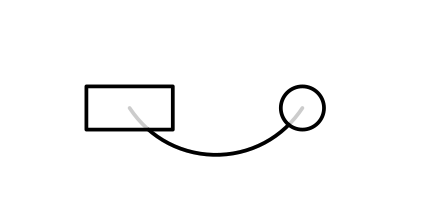The next section describes the 'gridGeometry' package in more detail and later sections provide some more examples of its use.

## 2. The 'gridGeometry' package

The 'gridGeometry' package provides functions for combining or transforming 'grid' grobs to create new grobs.

The `grid.polyclip` function, and the `polyclipGrob` function, allow us to combine grobs using operations supported by the `polyclip` function from the 'polyclip' package (Johnson and Baddeley, 2019) (which is an R interface to the C Clipper library; Johnson, 2019).

The following code demonstrates the four `polyclip` operations: intersection, union, minus, and xor. In each case, we combine an overlapping rectangle and circle (both drawn with thick black outlines) and the result is drawn as a filled grey shape with thick black outline.

```r <- rectGrob(.4, .4, .4, .4, gp=gpar(lwd=5))
c <- circleGrob(.6, .6, r=.2, gp=gpar(lwd=5))
```
```grid.draw(r)
grid.draw(c)
grid.polyclip(r, c, op="intersection",
gp=gpar(lwd=5, fill="grey"))
```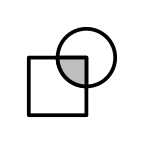```grid.draw(r)
grid.draw(c)
grid.polyclip(r, c, op="union",
gp=gpar(lwd=5, fill="grey"))
```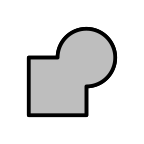```grid.draw(r)
grid.draw(c)
grid.polyclip(r, c, op="minus",
gp=gpar(lwd=5, fill="grey"))
```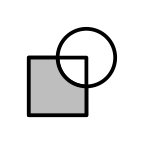```grid.draw(r)
grid.draw(c)
grid.polyclip(r, c, op="xor",
gp=gpar(lwd=5, fill="grey"))
```The 'gridGeometry' package is based on a fork of the 'polyclip' package that allows these operations to occur on (open) lines as well as (closed) polygons. The following code demonstrates the four `polyclip` operations when combining a Bezier curve with an overlapping circle. In each case, the curve and the circle are drawn with a thin black line and the result is drawn with a thick black line.

```l <- bezierGrob(c(.2, .4, .6, .8),
c(.2, .8, .8, .2))
c <- circleGrob(.6, .6, r=.2)
```
```grid.draw(l)
grid.draw(c)
grid.polyclip(l, c, op="intersection",
gp=gpar(lwd=5))
```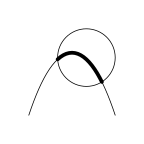```grid.draw(l)
grid.draw(c)
grid.polyclip(l, c, op="union",
gp=gpar(lwd=5))
```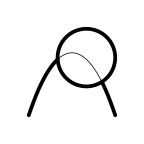```grid.draw(l)
grid.draw(c)
grid.polyclip(l, c, op="minus",
gp=gpar(lwd=5))
```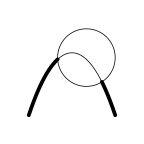```grid.draw(l)
grid.draw(c)
grid.polyclip(l, c, op="xor",
gp=gpar(lwd=5))
```The other high-level functions in 'gridGeometry' are `grid.trim` and `trimGrob`. These allow us to extract subsets of a line by specifying `from` and `to` arguments. The following code demonstrates its use by creating a variety of subsets of a Bezier curve. In each case, the complete curve is drawn as a thick grey line and the subsets are drawn as thick black lines.

```l <- bezierGrob(c(.2, .4, .6, .8),
c(.2, .8, .8, .2),
gp=gpar(col="grey", lwd=5))
```

The `from` and `to` arguments specify proportions of the length of the line. In the example below, we create a subset that starts 20% of the way along the curve and finishes 80% of the way along the curve.

```grid.draw(l)
grid.trim(l, .2, .8, gp=gpar(lwd=5))
```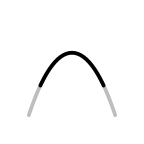Both `from` and `to` can be vectors, in which case multiple subsets are created. In the example below, we generate a subset from 20% to 40% and another subset from 60% to 80% of the way along the curve.

```grid.draw(l)
grid.trim(l, c(.2, .6), c(.4, .8), gp=gpar(lwd=5))
```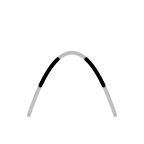Both `from` and `to` can also be negative, in which case the distance is measured from the end of the line. In the example below, we create a subset that starts 20% of the way along the curve and finishes 10% from the end of the curve.

```grid.draw(l)
grid.trim(l, .2, -.1, gp=gpar(lwd=5))
```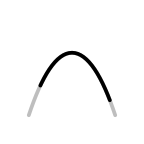Both `from` and `to` can be 'grid' units. In the example below, we create a subset that starts 5mm along the curve and finishes 1in from the end of the curve.

```grid.draw(l)
grid.trim(l, unit(5, "mm"), unit(-1, "in"), gp=gpar(lwd=5))
```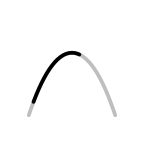When `from` and `to` are units, the "npc" coordinate system represents 0 to 1 along the line. This allows distances along the line to be specified as a combination of a proportions and units. In the example below, we create a subset that starts 20% of the way along the curve and finishes 5mm past 50% of the way along the curve.

```grid.draw(l)
grid.trim(l, .2, unit(.5, "npc") + unit(5, "mm"), gp=gpar(lwd=5))
```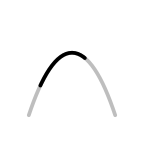There is also a `rep` argument that can be used to repeat `from` and `to` until the end of the line. The values are repeated by adding the `from` and `to` values onto the largest `to` value. In other words, the line that remains after the last `to` value is then used to generate more subsets using the original `from` and `to` values, and that process is repeated until there is no line left. In the example below, we create a subset that starts at 10% and finishes at 20%, then we create a subset that starts at 30% and finishes at 40%, and so on until a final subset that starts at 90% and finishes at 100%.

```grid.draw(l)
grid.trim(l, .1, .2, rep=TRUE, gp=gpar(lwd=5))
```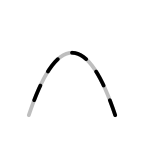## 3. Grob coordinates

The high-level functions like `grid.polyclip` begin with 'grid' grobs and generate 'grid' grobs as the result (and draw them). In between, they have to convert 'grid' grobs into a set of coordinates that can be fed to `polyclip` from the 'polyclip' package and then convert the result from `polyclip` back to a 'grid' grob. This section and the next section look at some functions that allow us to perform these conversions directly. This is useful if we want more control over the conversions between grobs and coordinates and if we want to perform further transformations on coordinates before converting back to grobs.

The conversion from a grob to a set of coordinates is made possible by the new function `grobCoords` in the 'grid' package. This function converts a grob into a list containing lists of x/y coordinates, with all values in inches relative to the current 'grid' viewport. There is also a `closed` argument to indicate whether we are looking for the coordinates of a closed shape or an open shape. This is straightforward for something like a single line. In the example below, we have a grob that specifies a line from the point (2in, 2in) to (3in, 3in) within the current viewport. Converting that to a set of coordinates in inches within the current viewport produces the expected result.

```l <- linesGrob(unit(2:3, "in"), unit(2:3, "in"))
lcoords <- grobCoords(l, closed=FALSE)
lcoords
```
```  []
[]\$x
 2 3

[]\$y
 2 3
```

However, some grobs generate many more coordinates. For example, in the case of a circle, the grob contains only centre and radius information, but the grob coordinates is a list of explicit points on the circumference of the circle. In the example below, we describe a circle centred at (2in, 2in) with a radius of 1in. The set of coordinates in inches within the current viewport consists of 100 (x, y) locations. (There is a argument `n` to control how many coordinates are generated for a circle).

```c <- circleGrob(2, 2, 1, default.units="in")
ccoords <- grobCoords(c, closed=TRUE)
ccoords
```
```  []
[]\$x
 3.000000 2.998027 2.992115 2.982287 2.968583 2.951057 2.929776 2.904827 2.876307 2.844328
 2.809017 2.770513 2.728969 2.684547 2.637424 2.587785 2.535827 2.481754 2.425779 2.368125
 2.309017 2.248690 2.187381 2.125333 2.062791 2.000000 1.937209 1.874667 1.812619 1.751310
 1.690983 1.631875 1.574221 1.518246 1.464173 1.412215 1.362576 1.315453 1.271031 1.229487
 1.190983 1.155672 1.123693 1.095173 1.070224 1.048943 1.031417 1.017713 1.007885 1.001973
 1.000000 1.001973 1.007885 1.017713 1.031417 1.048943 1.070224 1.095173 1.123693 1.155672
 1.190983 1.229487 1.271031 1.315453 1.362576 1.412215 1.464173 1.518246 1.574221 1.631875
 1.690983 1.751310 1.812619 1.874667 1.937209 2.000000 2.062791 2.125333 2.187381 2.248690
 2.309017 2.368125 2.425779 2.481754 2.535827 2.587785 2.637424 2.684547 2.728969 2.770513
 2.809017 2.844328 2.876307 2.904827 2.929776 2.951057 2.968583 2.982287 2.992115 2.998027

[]\$y
 2.000000 2.062791 2.125333 2.187381 2.248690 2.309017 2.368125 2.425779 2.481754 2.535827
 2.587785 2.637424 2.684547 2.728969 2.770513 2.809017 2.844328 2.876307 2.904827 2.929776
 2.951057 2.968583 2.982287 2.992115 2.998027 3.000000 2.998027 2.992115 2.982287 2.968583
 2.951057 2.929776 2.904827 2.876307 2.844328 2.809017 2.770513 2.728969 2.684547 2.637424
 2.587785 2.535827 2.481754 2.425779 2.368125 2.309017 2.248690 2.187381 2.125333 2.062791
 2.000000 1.937209 1.874667 1.812619 1.751310 1.690983 1.631875 1.574221 1.518246 1.464173
 1.412215 1.362576 1.315453 1.271031 1.229487 1.190983 1.155672 1.123693 1.095173 1.070224
 1.048943 1.031417 1.017713 1.007885 1.001973 1.000000 1.001973 1.007885 1.017713 1.031417
 1.048943 1.070224 1.095173 1.123693 1.155672 1.190983 1.229487 1.271031 1.315453 1.362576
 1.412215 1.464173 1.518246 1.574221 1.631875 1.690983 1.751310 1.812619 1.874667 1.937209
```

It is also possible for a single 'grid' grob to describe several shapes. In this case, the set of coordinates is a list with more than one component. In the example below, the grob describes two straight lines, one from (1in, 1in) to (2in, 2in) and one from (3in, 3in) to (4in, 4in). The result from `grobCoords` is a list of two sets of (x, y) coordinates.

```p <- polylineGrob(1:4, 1:4, default.units="in", id=rep(1:2, each=2))
grobCoords(p, closed=FALSE)
```
```  \$`1`
x y
1 1 1
2 2 2

\$`2`
x y
3 3 3
4 4 4
```

If we ask an open shape for closed coordinates, or a closed shape for open coordinates, we get an empty result. In the example below, we are asking an (open) line segment for closed coordinates.

```grobCoords(l, closed=TRUE)
```
```  \$x
 0

\$y
 0
```
```isEmptyCoords(grobCoords(l, closed=TRUE))
```
```   TRUE
```

The reason for distinguishing between open and closed coordinates is because it is possible to create a 'grid' grob that consists of both open and closed components. In the example below, we create a gTree grob that has an (open) line and a (closed) circle as its children. When we ask this gTree for closed coordinates, we get an empty result from the line, but a full set of coordinates from the circle.

```gt <- gTree(children=gList(l, c))
grobCoords(gt, closed=TRUE)
```
```  \$GRID.lines.34
\$GRID.lines.34\$x
 0

\$GRID.lines.34\$y
 0

\$GRID.circle.35
\$GRID.circle.35\$x
 3.000000 2.998027 2.992115 2.982287 2.968583 2.951057 2.929776 2.904827 2.876307 2.844328
 2.809017 2.770513 2.728969 2.684547 2.637424 2.587785 2.535827 2.481754 2.425779 2.368125
 2.309017 2.248690 2.187381 2.125333 2.062791 2.000000 1.937209 1.874667 1.812619 1.751310
 1.690983 1.631875 1.574221 1.518246 1.464173 1.412215 1.362576 1.315453 1.271031 1.229487
 1.190983 1.155672 1.123693 1.095173 1.070224 1.048943 1.031417 1.017713 1.007885 1.001973
 1.000000 1.001973 1.007885 1.017713 1.031417 1.048943 1.070224 1.095173 1.123693 1.155672
 1.190983 1.229487 1.271031 1.315453 1.362576 1.412215 1.464173 1.518246 1.574221 1.631875
 1.690983 1.751310 1.812619 1.874667 1.937209 2.000000 2.062791 2.125333 2.187381 2.248690
 2.309017 2.368125 2.425779 2.481754 2.535827 2.587785 2.637424 2.684547 2.728969 2.770513
 2.809017 2.844328 2.876307 2.904827 2.929776 2.951057 2.968583 2.982287 2.992115 2.998027

\$GRID.circle.35\$y
 2.000000 2.062791 2.125333 2.187381 2.248690 2.309017 2.368125 2.425779 2.481754 2.535827
 2.587785 2.637424 2.684547 2.728969 2.770513 2.809017 2.844328 2.876307 2.904827 2.929776
 2.951057 2.968583 2.982287 2.992115 2.998027 3.000000 2.998027 2.992115 2.982287 2.968583
 2.951057 2.929776 2.904827 2.876307 2.844328 2.809017 2.770513 2.728969 2.684547 2.637424
 2.587785 2.535827 2.481754 2.425779 2.368125 2.309017 2.248690 2.187381 2.125333 2.062791
 2.000000 1.937209 1.874667 1.812619 1.751310 1.690983 1.631875 1.574221 1.518246 1.464173
 1.412215 1.362576 1.315453 1.271031 1.229487 1.190983 1.155672 1.123693 1.095173 1.070224
 1.048943 1.031417 1.017713 1.007885 1.001973 1.000000 1.001973 1.007885 1.017713 1.031417
 1.048943 1.070224 1.095173 1.123693 1.155672 1.190983 1.229487 1.271031 1.315453 1.362576
 1.412215 1.464173 1.518246 1.574221 1.631875 1.690983 1.751310 1.812619 1.874667 1.937209
```

If we ask the gTree for open coordinates, we get coordinates from the line and an empty result from the circle.

```grobCoords(gt, closed=FALSE)
```
```  \$GRID.lines.34
\$GRID.lines.34\$x
 2 3

\$GRID.lines.34\$y
 2 3

\$GRID.circle.35
\$GRID.circle.35\$x
 0

\$GRID.circle.35\$y
 0
```

Once we have grob coordinates, we can call functions like `polyclip` from the 'polyclip' package to combine the coordinates. The result is a new set of coordinates. In the example below, we intersect the line with the circle and the result is a truncated line (up to the edge of the circle). The `closed` argument (which has been added in the fork of the 'polyclip' package) tells `polyclip` whether its first argument is open or closed (`polyclip` requires the second argument to always be a closed shape).

```polyclip(lcoords, ccoords, closed=FALSE)
```
```  []
[]\$x
 2.000000 2.706758

[]\$y
 2.000000 2.706758
```

We can also use a set of coordinates in other calculations. For example, the following code draws a variable-width line around the circumference of the circle (using the 'vwline' package; , Murrell, 2018). The value of `grobCoords` here is to convert a circle specification into (essentially) a series of straight line segments, which we can then use with the `grid.vwcurve` function to draw a variable-width line.

```library(vwline)
cx <- ccoords[]\$x
cy <- ccoords[]\$y
grid.vwcurve(cx, cy, default.units="in",
w=seq(0, 1, length.out=length(cx)))
```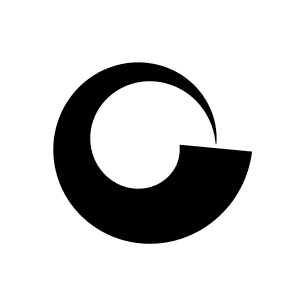The 'gridGeometry' package also defines a generic version of the `polyclip` function, with `polyclip::polyclip` as the default and a new method for 'grid' grobs. This means that we can generate coordinates for a combination of grobs directly. The following code sends `polyclip` the original grobs rather than their coordinates. The result is still a set of coordinates.

```polyclip(l, c, closed=FALSE)
```
```  []
[]\$x
 2.000000 2.706758

[]\$y
 2.000000 2.706758
```

There is also a `polyclip` method for gPaths, so that it is possible to refer by name to grobs that have already been drawn. The following code demonstrates this by drawing a line named "l" and a circle named "c" and then calling polyclip to produce coordinates for the intersection of the grobs named "l" and "c".

```grid.lines(unit(2:3, "in"), unit(2:3, "in"), name="l")
grid.circle(2, 2, 1, default.units="in", name="c")
polyclip("l", "c", closed=FALSE)
```
```  []
[]\$x
 2.000000 2.706758

[]\$y
 2.000000 2.706758
```

There is also a low-level function that underlies the `grid.trim` and `trimGrob` functions. The `trim` function takes either a grob, or a set of coordinates, and generates a new set of coordinates, in this case representing one or more subsets of the original grob coordinates. For example, in the code below, we generate an X-spline grob that starts at (0in, 0in), ends at (2in, 01in) and passes through (1in, 1in) and then we call `trim` to calculate the coordinates of two subsets of the X-spline curve: the first half of the curve and the last half of the curve.

```xg <- xsplineGrob(0:2, c(0, 1, 0), default.units="in", shape=-1)
```
```xcoords <- trim(xg, from=0:1/2, to=1:2/2)
xcoords
```
```  []
[]\$x
 0.000 0.000 0.003 0.010 0.023 0.043 0.070 0.103 0.142 0.186 0.235 0.287 0.342 0.401 0.463 0.529
 0.600 0.676 0.759 0.848 0.942 1.000

[]\$y
 0.000 0.001 0.006 0.020 0.045 0.082 0.132 0.192 0.260 0.335 0.414 0.493 0.572 0.648 0.720 0.787
 0.848 0.901 0.945 0.978 0.997 1.000

[]
[]\$x
 1.000 1.000 1.096 1.189 1.275 1.355 1.429 1.498 1.562 1.623 1.680 1.734 1.785 1.832 1.875 1.911
 1.942 1.966 1.983 1.993 1.998 2.000 2.000

[]\$y
 1.000 1.000 0.991 0.966 0.929 0.881 0.824 0.761 0.692 0.618 0.541 0.461 0.382 0.305 0.232 0.166
 0.110 0.066 0.034 0.014 0.003 0.000 0.000
```

The following code draws the two halves of the curve with different line widths.

```pushViewport(viewport(width=2/3, height=2/3))
grid.lines(xcoords[]\$x, xcoords[]\$y, default.units="in",
gp=gpar(lwd=3, lineend="butt"))
grid.lines(xcoords[]\$x, xcoords[]\$y, default.units="in",
gp=gpar(lwd=10, lineend="butt"))
```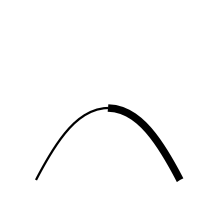## 4. Grobs from coordinates

If we have worked directly in the world of grob coordinates, using functions from the previous section, we usually want to get back to the world of grobs, so that we can draw the final result.

We have seen a couple of examples of drawing shapes from coordinates by calling 'grid' functions and taking into account that the coordinates in are inches. The 'gridGeometry' package provides several convenience functions that make this job a little less work: `xyListLine`, `xyListPath` and `xyListPolygon`.

The main benefit of these functions is that they automatically assume inches as the coordinate system and they automatically handle multiple shapes. For example, in the following code we subtract a thin rectangle from a circle using `polyclip` directly, so the result is a set of coordinates.

```coords <- polyclip(c, rectGrob(width=.2), op="minus")
coords
```
```  []
[]\$x
 1.600000 1.574221 1.518246 1.464173 1.412215 1.362576 1.315453 1.271031 1.229487 1.190983
 1.155672 1.123693 1.095173 1.070224 1.048943 1.031417 1.017713 1.007885 1.001973 1.000000
 1.001973 1.007885 1.017713 1.031417 1.048943 1.070224 1.095173 1.123693 1.155672 1.190983
 1.229487 1.271031 1.315453 1.362576 1.412215 1.464173 1.518246 1.574221 1.600000

[]\$y
 2.915983 2.904827 2.876307 2.844328 2.809017 2.770513 2.728969 2.684547 2.637424 2.587785
 2.535827 2.481754 2.425779 2.368125 2.309017 2.248690 2.187381 2.125333 2.062791 2.000000
 1.937209 1.874667 1.812619 1.751310 1.690983 1.631875 1.574221 1.518246 1.464173 1.412215
 1.362576 1.315453 1.271031 1.229487 1.190983 1.155672 1.123693 1.095173 1.084017

[]
[]\$x
 2.425779 2.481754 2.535827 2.587785 2.637424 2.684547 2.728969 2.770513 2.809017 2.844328
 2.876307 2.904827 2.929776 2.951057 2.968583 2.982287 2.992115 2.998027 3.000000 2.998027
 2.992115 2.982287 2.968583 2.951057 2.929776 2.904827 2.876307 2.844328 2.809017 2.770513
 2.728969 2.684547 2.637424 2.587785 2.535827 2.481754 2.425779 2.400000 2.400000

[]\$y
 1.095173 1.123693 1.155672 1.190983 1.229487 1.271031 1.315453 1.362576 1.412215 1.464173
 1.518246 1.574221 1.631875 1.690983 1.751310 1.812619 1.874667 1.937209 2.000000 2.062791
 2.125333 2.187381 2.248690 2.309017 2.368125 2.425779 2.481754 2.535827 2.587785 2.637424
 2.684547 2.728969 2.770513 2.809017 2.844328 2.876307 2.904827 2.915983 1.084017
```

The `xyListPolygon` function automatically copes with the fact that the result is two separate shapes and generates a polygon grob that we can draw.

```grid.draw(xyListPolygon(coords,
gp=gpar(lwd=5, fill="grey")))
```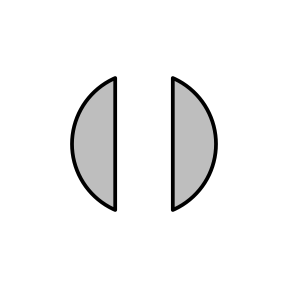It is important to note that a call to the `grobCoords` function is when grob coordinates get converted to inches within the current 'grid' viewport. This means that the coordinates that are generated are relative to the viewport in effect when `grobCoords` is called.

In the code below, we generate a rectangle grob and call `grobCoords` to calculate its coordinates. We then draw the rectangle grob and a polygon based on its coordinates, in the same viewport that was in effect when we calculated the coordinates, so the rectangle (grey) and the polygon (dotted black) match up.

```r <- rectGrob(width=.8, height=.8, gp=gpar(lwd=5, col="grey"))
coords <- grobCoords(r, closed=TRUE)
grid.draw(r)
grid.draw(xyListPolygon(coords,
gp=gpar(lwd=5, lty="dotted")))
```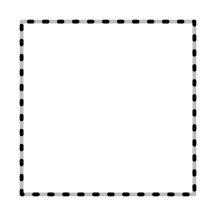Next, we push a viewport (in the central quarter of the image) and draw the grob and the polygon within this viewport. This is not the viewport that the coordinates were calculated within, so the polygon (red dotted) does not match the rectangle (inner grey).

```pushViewport(viewport(width=.5, height=.5))
grid.draw(r)
grid.draw(xyListPolygon(coords,
gp=gpar(lwd=5, lty="dotted", col="red")))
```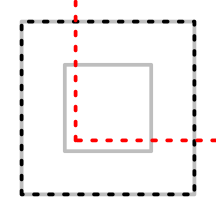Finally, we call `grobCoords` again, this time within the new viewport, and now the rectangle (inner grey) and the polygon (dotted blue) match up again.

```grid.draw(xyListPolygon(grobCoords(r, closed=TRUE),
gp=gpar(lwd=5, lty="dotted", col="blue")))
```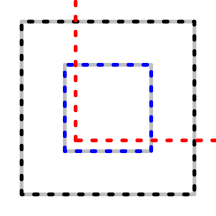On the other hand, the `grobCoords` function automatically takes into account any `vp` setting (and for gTrees any `childrenvp` settings). For example, in the following code we create a rectangle grob with a `vp` argument that means that the rectangle will be drawn in the central quarter of the image. If we draw the rectangle (grey) and call `grobCoords` and draw a polygon from the resulting coordinates (dotted black) in the same viewport, we get a matching result.

```r <- rectGrob(width=.8, height=.8,
gp=gpar(lwd=5, col="grey"),
vp=viewport(width=.5, height=.5))
grid.draw(r)
grid.draw(xyListPolygon(grobCoords(r, closed=TRUE),
gp=gpar(lwd=5, lty="dotted")))
```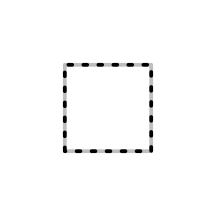It is also important to note that the coordinates generated by `grobCoords` are "neutral" in the sense that they do not retain any information about whether they were generated from a closed or open shape, or any details like fill rules for more complex shapes. This means that we can impose semantics on the coordinates that are different from the semantics of the original grob. As an example, consider the following lines grob.

```lg <- linesGrob(c(.2, .2, .8, .8, .4, .6),
c(.2, .8, .8, .2, .6, .6),
gp=gpar(lwd=3))
grid.draw(lg)
```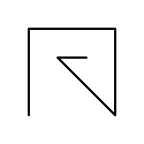We can generate coordinates from this line and then a grob from the coordinates and get a filled shape. We must specify `closed=FALSE` for the call to `grobCoords` or we will get an empty result, but once we have coordinates we can use them however we wish. In this case, by passing the coordinates to the `xyListPath` function, we treat the coordinates as a closed path shape.

```lgcoords <- grobCoords(lg, closed=FALSE)
lgpath <- xyListPath(lgcoords, rule="evenodd", gp=gpar(fill="black"))
grid.draw(lgpath)
```It is also possible to dictate how coordinates are interpreted when we call the `polyclip` function. The following code treats the line coordinates as a closed shape to intersect those coordinates with the coordinates for a circle. We specify `closed=TRUE` in the call to `polyclip` so that it interprets the coordinates for the line as a closed shape.

```coords <- polyclip(lgcoords,
grobCoords(circleGrob(.5, .5, .3), closed=TRUE),
closed=TRUE,
fillA="evenodd")
```

The result of `polyclip` is a new set of coordinates and we can use them to draw an open line ...

```grid.draw(xyListLine(coords, gp=gpar(lwd=3)))
```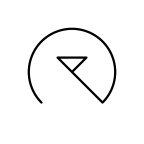... or a closed path ...

```grid.draw(xyListPath(coords, gp=gpar(fill="black")))
```## 5. Grobs versus coordinates

This section looks at some important differences between 'grid' grobs and sets of coordinates.

The `grobCoords` function generates points on the boundary of a 'grid' grob based on the basic description of the shape of the grob. This does not take into account things like the colour of the grob, whether the grob is filled in or not, or, more importantly, the thickness of the line used to draw the boundary of the grob.

The following code demonstrates this difference by subtracting a rectangle from a circle, both of which are drawn with thick lines. The resulting intersection is drawn as a thin red line. Notice that only the basic circle shape has been subtracted from the rectangle, ignoring the thickness of the circle border and ignoring the thickness of the rectangle border.

```r <- rectGrob(.4, .4, .4, .4, gp=gpar(lwd=10))
c <- circleGrob(.6, .6, r=.2, gp=gpar(lwd=10))
```
```grid.draw(r)
grid.draw(c)
grid.polyclip(r, c, op="minus",
gp=gpar(col="red"))
```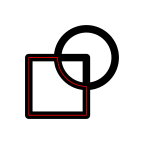If we draw the result with the same line thickness as the circle and the rectangle, the result looks even worse (it looks like only the interior of the circle has been removed from the rectangle) ...

```grid.draw(r)
grid.draw(c)
grid.polyclip(r, c, op="minus",
gp=gpar(col="red", lwd=10))
```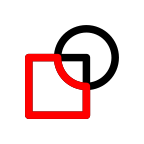If we actually want to subtract the outline of the drawn border of the circle, we need coordinates that describe that outline. One way to do that is with the 'vwline' package, which generates a polygon or path to represent a line (potentially with a variable width).

The following code calls `grobCoords` to get the coordinates of the rectangle and the circle. We then generate a variable-width line (with a constant width) based on the circle coordinates. Next, we generate the coordinates for that variable-width line (which has coordinates for both the inside and outside of the circle border). Finally, we generate a polygon by subtracting the second set of coordinates for the variable-width line from the rectangle coordinates.

```rc <- grobCoords(r, closed=TRUE)
cc <- grobCoords(c, closed=TRUE)[]
vwc <- vwcurveGrob(cc\$x, cc\$y, default.units="in", w=unit(10/96, "in"),
open=FALSE)
vwcc <- grobCoords(vwc, closed=TRUE)
result <- xyListPolygon(polyclip(rc, vwcc, "minus"), gp=gpar(col="green"))
```

The figure below shows the original rectangle, the variable-width line and result of the subtraction (in green). Many other variations on this result are possible by playing directly with coordinates of grobs.

```grid.draw(r)
grid.draw(vwc)
grid.draw(result)
```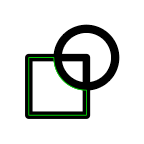## 6. Examples

One simple application of 'gridGeometry' is to generate a polygonal shape that is difficult to describe directly, as in the original line between two graph nodes at the start of this document.

The code below shows a closed-shape version of this sort of task (for a fairly arbitrary shape). The goal is a rectangle with circular holes punched in it, including semicircular holes at the edges (the filled grey region below). This shape could be described explicitly as a path in 'grid', but it is much easier to describe a rectangle and a series of circles and subtract the latter from the former.

```r <- rectGrob(width=.6, height=.4, gp=gpar(lwd=5))
c <- circleGrob(x=1:4/5, r=unit(5, "mm"), gp=gpar(lwd=5))
p <- polyclipGrob(r, c, op="minus",
gp=gpar(fill=rgb(0,0,0,.5), lwd=5))
grid.draw(r)
grid.draw(c)
grid.draw(p)
```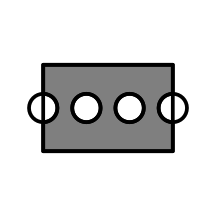The next example shows a similar construction, but in a larger context (at the risk of inviting the attention of the chart-junk police). The overall plot is a 'lattice' barchart (Sarkar, 2008) of counts of movies in different genres (Wickham, 2015). We have provided a panel function that, after drawing the normal bars, constructs a "flim strip" shape by substracting lots of small rectangles and circles from the normal bars. This demonstrates the use of a gPath as the first argument to `grid.polyclip` which, by default, replaces the named grob with the result of the polyclip operation (by default retaining the graphical parameters of the original grob).

```library(ggplot2movies)
counts <- apply(movies[-(1:17)], 2, sum)
filmstrip <- function(x, y, box.width=2/3, ...) {
panel.barchart(x, y, box.width, ...)
w <- convertWidth(unit(1, "npc"), "cm", valueOnly=TRUE)
hsteps <- seq(-.1, w, .7)
hx <- rep(hsteps, length(x))
hy <- rep(y, each=length(hsteps))
holes <- rectGrob(x=unit(hx, "cm"), y=unit(hy, "native"),
width=unit(.5, "cm"), height=unit(.7, "cm"),
just="left")
esteps <- seq(.1, w, .2)
ex <- rep(esteps, 2*length(x))
ey <- rep(c(as.numeric(y) + .4*box.width,
as.numeric(y) - .4*box.width),
each=length(esteps))
edges <- circleGrob(unit(ex, "cm"), unit(ey, "native"),
r=unit(.5, "mm"))
grid.polyclip("plot_01.barchart.rect.panel.1.1",
gList(holes, edges), "minus")
}
library(lattice)
barchart(sort(counts),
panel=function(x, y, ...) {
filmstrip(x, y, ...)
})
```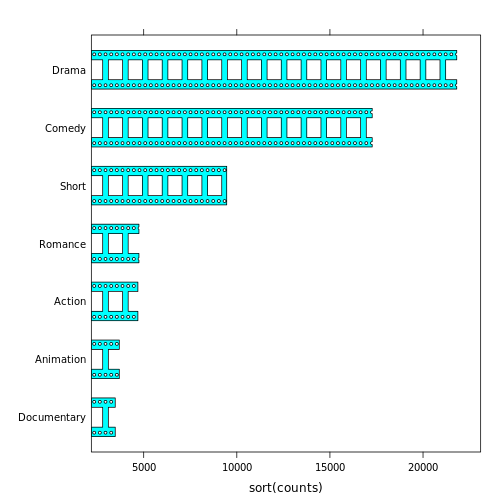The next example demonstrates an application of the `trim` function. To motivate the problem, we first draw a Bezier curve with an arrow head with standard 'grid' functions.

```x <- c(.2, .5, .5, .8)
y <- c(.2, 0, 1, .8)
grid.bezier(x, y, gp=gpar(lwd=5, fill=NA),
arrow=arrow(angle=20, length=unit(1, "cm"), type="closed"))
```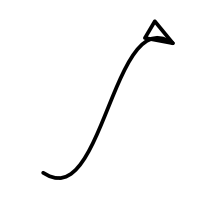There are two problems with the result above: the arrow head is drawn with the same graphical parameters as the line, so it is thick with rounded corners; and the orientation of the arrow head is based on the angle of the last straight line segment in the "curve", so it looks silly (because the end of the line has high curvature).

We are going to improve the arrow head by drawing the curve in separate parts. First, we get the coordinates for the line and split those into three parts: the last 10mm of the curve; 8mm from the end to 12mm from the end of the curve; and all but the last 10mm of the curve.

```bg <- bezierGrob(x, y, gp=gpar(lwd=5, col=rgb(0,0,0,.2)))
pts <- grobCoords(bg, closed=FALSE)
segs <- trim(pts,
from=unit(c(1, -8, -10), c("npc", "mm", "mm")),
to=unit(c(-10, -12, 0), c("mm", "mm", "npc")))
```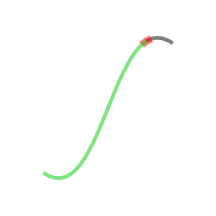Now we can draw most of the curve as a normal line and then add a variable-width line based on the last 10mm of the curve to produce an arrow head that follows the curve.

```line <- xyListLine(segs, gp=gpar(lwd=5))
arrow <- offsetXsplineGrob(segs[]\$x, segs[]\$y, default.units="in",
shape=1,
w=widthSpline(unit(c(6, 0), "mm")))
grid.draw(line)
grid.draw(arrow)
```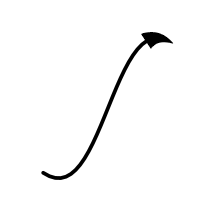The following code takes this a step further and adds in a bit of constructive geometry. This time we draw all but the last 8mm of the curve as a normal line. We then create two variable-width lines, one based on the last 10mm of the curve as before, and a second based on the section of the curve from 8mm to 12mm from the end. We make the latter variable-width line expand much faster than the former (the two variable-width lines are shown in semitransparent red and semitransparent blue below).

```line <- xyListLine(segs[2:3], gp=gpar(lwd=5))
arrow1 <- offsetXsplineGrob(segs[]\$x, segs[]\$y, default.units="in",
shape=1,
w=widthSpline(unit(c(6, 0), "mm")),
gp=gpar(fill=rgb(1,0,0,.5)))
arrow2 <- offsetXsplineGrob(segs[]\$x, segs[]\$y, default.units="in",
shape=1,
w=widthSpline(unit(c(12, 0), "mm")),
gp=gpar(fill=rgb(0,0,1,.5)))
grid.draw(line)
grid.draw(arrow1)
grid.draw(arrow2)
```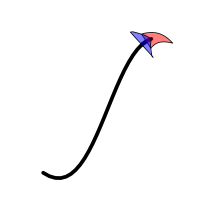We construct a final arrow head by subtracting one variable-width line from the other to produce a curve with a notched arrow head that follows the curve (and has nice pointy corners).

```arrow <- polyclipGrob(arrow1, arrow2, "minus",
gp=gpar(fill="black"))
grid.draw(line)
grid.draw(arrow)
```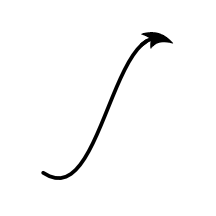That may seem like a lot of work, but because it is code-based, this approach could easily be wrapped into a function to simplify reuse and enhance generality.

## 7. Discussion

This report has described two new graphics facilities in R. One is the `grobCoords` function in the 'grid' package, which can be used to convert 'grid' grobs into sets of coordinates that describe the shape of the grob. The other is the 'gridGeometry' package, which provides functions for combining grobs, for example, calculating the intersection of two grobs.

It might reasonably be asked what purpose the 'gridGeometry' package is really serving. The `grobCoords` function performs the conversion from grobs into coordinates and the 'polyclip' package does all of the hard work of combining sets of coordinates. What does 'gridGeometry' add to that?

The answer is, mostly, just convenience. Most of the functions in the 'gridGeometry' package are there to provide an interface to the process of combining 'grid' grobs. However, the `grid.trim` function for generating subsets of lines is also a new contribution.

What is the value of having this convenient interface to a geometry engine? The main idea is that this provides access to a new tool. For example, prior to having this tool, we might never have thought of turning a bar plot into a film strip. Some might argue that we should never have that thought and that that is not a good thought, but the important point is that new tools help us to think of new ways of doing things. New tools help us to find new solutions to problems.

### Related work

There are several other R packages that make use of the geometry engine that is provided by the 'polyclip' package. For example, 'eulerr' (Larsson, 2019) and 'SubgrPlots' (Ballarini and Chiu, 2018) use 'polyclip' for drawing (variations of) venn diagrams. The 'maptools' package (Bivand and Lewin-Koh, 2019) uses 'polyclip' to clip map regions (when they wrap from left-edge to right-edge of map, or vice versa) and 'deldir' (Turner, 2019) uses 'polyclip' for complex clipping of voronoi tesselations. The 'ggforce' (Pedersen, 2019) and 'ggraph' (Pedersen, 2018) packages use 'polyclip' for tasks very similar to those demonstrated in this report (cutting edges between nodes and modifying or combining shapes), but provides these facilities as 'ggplot2' extensions rather than at the level of 'grid'. The 'spatstat' package (Baddeley et al., 2015) uses 'polyclip' for all sorts of things, including polygon offsets, polyline offsets, and polygon intersection. The main difference betweeen 'gridGeometry' and these other packages is in how the shapes that are to be combined are generated. The contribution of 'gridGeometry' (and `grid::grobCoords`) is to add the ability to generate shapes from 'grid' grobs (which includes anything that is drawn by packages that are built on top of 'grid').

### Limitations

The usual issue of speed (or slowness) is a problem for the 'gridGeometry' package. This is not the fastest possible way to perform constructive geometry. However, in some applications and for some users, the convenience may be worth the wait.

Another important limitation of 'gridGeometry', or more specifically, the `grobCoords` function, is that it is not (currently) possible to generate a set of coordinates for all 'grid' grobs. It is perhaps not surprising that raster grobs, "clip" grobs, and "null" grobs generate an empty set of coordinates, but, in addition, text grobs and point grobs generate an empty set of coordinates. These gaps may be the subject of future work.

## 8. Technical requirements

The examples and discussion in this document relate to version 0.2-0 of the 'gridGeometry' package, version 1.10-0 of the 'polyclip' package (to allow for open paths), and R version 3.6.0 (for the `grobCoords` function).

This report was generated within a Docker container (see Resources section below).

## 9. Resources

• The raw source file for this report, a valid XML transformation of the source file, a 'knitr' document generated from the XML file, two R files and the bibtex file that are used to generate the table of contents and reference sections, two XSL files and an R file that are used to transform the XML to the 'knitr' document, and a Makefile that contains code for the other transformations and coordinates everything. These materials are also available on github.
• This report was generated within a Docker container. The Docker command to build the report is included in the Makefile above. The Docker image for the container is available from Docker Hub; alternatively, the image can be rebuilt from its Dockerfile.

## How to cite this document

Murrell, P. (2019). "A Geometry Engine Interface for 'grid'" Technical Report 2019-01, Department of Statistics, The University of Auckland. Version 4. [ bib | DOI | http ]

## 10. References

Baddeley, A., Rubak, E., and Turner, R. (2015). Spatial Point Patterns: Methodology and Applications with R. Chapman and Hall/CRC Press, London. [ bib | http ]
[Ballarini and Chiu, 2018]
Ballarini, N. and Chiu, Y.-D. (2018). SubgrPlots: Graphical Displays for Subgroup Analysis in Clinical Trials. R package version 0.1.0. [ bib | http ]
[Bivand and Lewin-Koh, 2019]
Bivand, R. and Lewin-Koh, N. (2019). maptools: Tools for Handling Spatial Objects. R package version 0.9-5. [ bib | http ]
[Johnson, 2019]
Johnson, A. (2019). Clipper - an open source freeware library for clipping and offsetting lines and polygons. http://www.angusj.com/delphi/clipper.php. Accessed: 2019-02-27. [ bib ]
Johnson, A. and Baddeley, A. (2019). polyclip: Polygon Clipping. R package version 1.10-0. [ bib | http ]
Larsson, J. (2019). eulerr: Area-Proportional Euler and Venn Diagrams with Ellipses. R package version 5.1.0. [ bib | http ]
[Murrell, 2017]
Murrell, P. (2017). Variable-width lines in R. Technical Report 2017-01, Department of Statistics, The University of Auckland. Version 1. [ bib | DOI | http ]
[Murrell, 2018]
Murrell, P. (2018). vwline: Draw variable-width lines. R package version 0.2-1. [ bib ]
[Murrell, 2019]
Murrell, P. (2019). gridGeometry: Polygon Geometry in grid. R package version 0.1-0. [ bib ]
[Pedersen, 2018]
Pedersen, T. L. (2018). ggraph: An Implementation of Grammar of Graphics for Graphs and Networks. R package version 1.0.2. [ bib | http ]
[Pedersen, 2019]
Pedersen, T. L. (2019). ggforce: Accelerating 'ggplot2'. R package version 0.2.0. [ bib | http ]
[R Core Team, 2018]
R Core Team (2018). R: A Language and Environment for Statistical Computing. R Foundation for Statistical Computing, Vienna, Austria. [ bib | http ]
[Sarkar, 2008]
Sarkar, D. (2008). Lattice: Multivariate Data Visualization with R. Springer, New York. ISBN 978-0-387-75968-5. [ bib | http ]
[Turner, 2019]
Turner, R. (2019). deldir: Delaunay Triangulation and Dirichlet (Voronoi) Tessellation. R package version 0.1-16. [ bib | http ]
[Urbanek, 2014]
Urbanek, S. (2014). jpeg: Read and write JPEG images. R package version 0.1-8. [ bib | http ]
[Wickham, 2015]
Wickham, H. (2015). ggplot2movies: Movies Data. R package version 0.0.1. [ bib | http ]
[Wickham, 2016]
Wickham, H. (2016). ggplot2: Elegant Graphics for Data Analysis. Springer-Verlag New York. [ bib | http ]